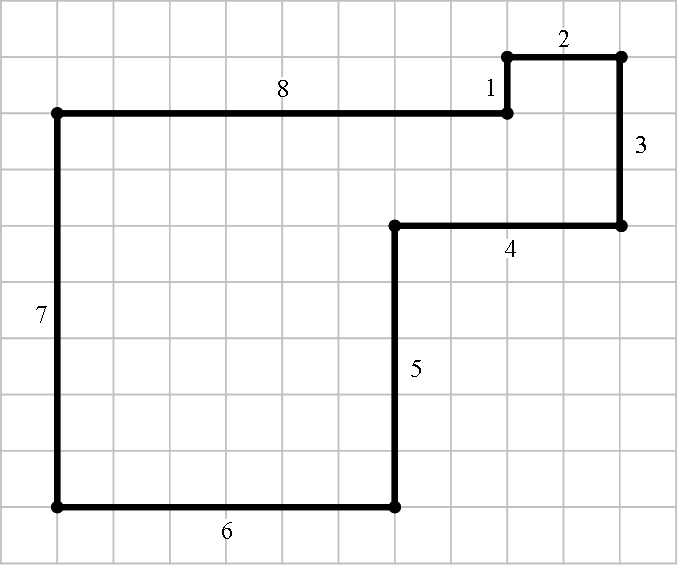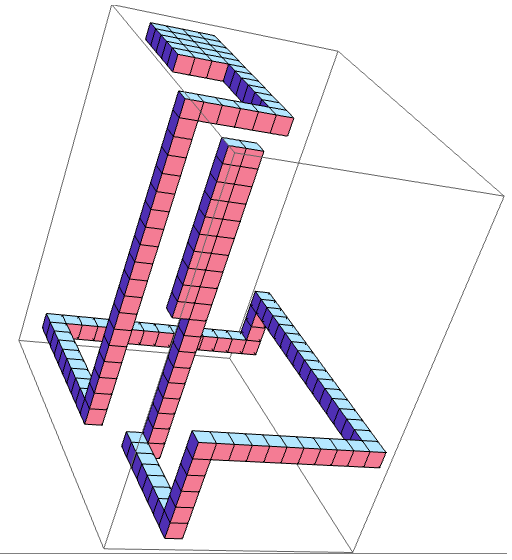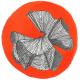## Golygons and golyhedra

A most delightful way to procrastinate is to attempt the unsolved problems on MathOverflow. Especially rewarding is when you actually end up solving such a problem, or when you learn something in the process, or both. Last night I learnt the terms golygon (coined by Lee Sallows) and golyhedron (coined by Joseph O’Rourke before their existence had even been established), eventually providing an example of the latter after a reasonable amount of work.

### Golygons

A golygon is a lattice polygon where all angles are right-angles, and the sides have consecutive integer lengths {1, 2, 3, …, n}. The simplest example is the following octagon:Notice that the edges, in cyclic order, are {1, 2, 3, 4, 5, 6, 7, 8}. The Wikipedia article mentions the interesting fact that this is capable of tiling the plane, and indeed this is possible: two copies of the tile, rotated 180°, can be slotted together to form a unit cell which can tessellate the plane periodically.One problem is to count the number of golygons with n edges. If we allow self-intersections, then this amounts to counting the number of solutions to the following equations:

• ± 1 ± 3 ± 5 ± … ± (n − 1) = 0
• ± 2 ± 4 ± 6 ± … ± n = 0

Obviously, n must be even, since we alternate between vertical and horizontal moves. We also require 2 + 4 + 6 + … + n to be a multiple of 4, so as to be able to partition it into two sums of even numbers; this means that n is congruent to either 6 or 0 (modulo 8). Finally, we require 1 + 3 + 5 + … + (n − 1) to be even, so n must be divisible by 4. Consequently, every golygon has n = 8k edges for some positive integer k. These are enumerated by this sequence, which uses generating functions.

Forbidding self-intersections makes the problem much harder, as we are then counting self-avoiding walks of a particular nature. This problem is, in general, very difficult.

### Golyhedra

This was, of course, merely a warm-up for explaining the three-dimensional generalisation, a golyhedron. In defining a golyhedron, Joseph O’Rourke first imposed some suitable regularity conditions:

• All vertices of P belong to the three-dimensional integer lattice$\mathbb{Z}^3$;
• All edges of P are parallel to a coordinate axis;
• All faces of P are simply-connected;
• The boundary ∂P is topologically a sphere.

‘Regularity conditions’ just mean that we restrict attention to objects that are quite nice. For example, in differential geometry one considers surfaces that are continuously differentiable; in complex analysis, we mostly consider holomorphic functions on open subsets of the complex plane (or some other Riemann surface).

Naturally, we still have to impose a further condition, namely the interesting one. The area sequence of P is the sorted list of the areas of the faces of P. He proceeds to give a very helpful example:If we did this for the golygon at the beginning of the page, and modified the definitions to apply to two-dimensional polygons, then it would have a length sequence of {1, 2, 3, 4, 5, 6, 7, 8}. This motivates the definition of a golyhedron:

• A golyhedron is a polyhedron P obeying the four aforementioned regularity conditions and possessing area sequence {1, 2, 3, … , n} for some n.

After some experimentation, Joseph O’Rourke conjectured that no such golyhedra exist. It is difficult, for example, to avoid having lots of faces with small area. Somewhat out of the blue, I was inspired by this orthogonal polyhedron from David Eppstein’s blog post.Eppstein mainly focused on the skeleton of the polyhedron, which is a vertex-transitive 3-regular graph with 24 vertices and 36 edges. However, for this problem we are more interested in the faces of the polyhedron, of which there are twelve. They are all L-shaped, and come in pairs whose areas differ by 2.

Obviously, this toroidal polyhedron fails for multiple reasons. Firstly, its surface is not topologically a sphere. Secondly, it is far from being a golyhedron, since the symmetry means that areas are repeated. However, it seemed quite promising, since it might be possible to realise a golyhedron using a similar local structure. In particular, I envisaged something like this:Ignore, for the moment, the fact that this actually is a golyhedron. It is simply-connected, and principally consists of a long tortuous chain of unit cubes, which changes direction like so:

down, left, forwards, up, right, backwards, down, left, forwards, up, right, backwards

This cycles through the directions in the same way as Eppstein’s doughnut, so we end up with a bunch of L-shaped faces occurring in pairs whose areas differ by 2. If we simply truncated the ends of the chain, we would have two faces of area 1, so I instead terminated the ends with club-foot constructions.

I decided that it would be helpful to build the club-feet first, and then attempt to connect them together using a chain. Note that the opposite L-shapes have areas differing by 2, so I needed the areas unused by the club feet to be partitionable into pairs of that form. I also wanted the club feet to include the faces of small area, so that the L-shapes could be nice and large. Subject to these constraints, I found the following possible sequence:The 14 red numbers are those covered by the club feet. The remaining 18 black numbers can be matched into 9 pairs as shown above; these will be represented by pairs of L-shapes. We will define the abstract length of an edge in the chain to be n − 1, where n is the number of cubes. Then the L-shapes induced by consecutive edges of length n and m will have areas of n + m ± 1. Consequently, we obtain the following system of equations:

• 1 + a = x1
• a + b = x2
• b + c = x3
• c + d = x4
• d + e = x5
• e + f = x6
• f + g = x7
• g + h = x8
• h + 5 = x9

where all of the variables are positive integers, and {x1, …, x9} are (in some order) {8, 12, 15, 16, 19, 22, 23, 26, 27}. By subtracting the even-indexed xs from the odd-indexed xs, we obtain:

• x1 − x2 + x3 − x4 + x5 − x6 + x7 − x8 + x9 = 6

And of course, the sum of the xs is equal to the sum of {8, 12, 15, 16, 19, 22, 23, 26, 27} (the mean values of each pair), namely 168, giving another linear equation:

• x1 + x2 + x3 + x4 + x5 + x6 + x7 + x8 + x9 = 168

A change of basis gives these two simpler equations:

• x1 + x3 + x5 + x7 + x9 = 87
• x2 + x4 + x6 + x8 = 81

A possible solution to this is the partition {8, 15, 19, 22, 23} ∪ {12, 16, 26, 27}. We then need to choose the order of the terms within each part to accomplish each of the following goals:

• a, b, …, h are all positive;
• The resulting polyhedron does not self-intersect.

After experimentation, I settled on the order (8, 12, 15, 26, 19, 16, 22, 27, 23) for the xs, which gives edge lengths (a, b, …, h) = (7, 5, 10, 16, 3, 13, 9, 18). The resulting golyhedron is shown below:So, we have a golyhedron with 32 faces. What is the smallest number of faces possible?

Any golyhedron must have an even surface area, so the number of faces must be congruent to either 0 or 3 (modulo 4). There are also no 7- or 8-faced golyhedra, since any such polyhedron must (by the pigeonhole principle) have a plane (either xy, yz or zx), parallel to which there are only two faces, which must therefore both have equal area.

Consequently, we can bound the minimum number of faces of a golyhedron between 11 and 32. Can you improve upon those bounds?

If so, either comment here or (if you have a MO account) the original question.

This entry was posted in Uncategorized. Bookmark the permalink.

### 10 Responses to Golygons and golyhedra

1.Michael Wang says:

This is not an improvement upon those bounds, but merely a (rather useless) observation:
David Eppstein’s polyhedron has been constructed in real life as a sculpture, “Cubo Vazado” (Emptied Cube), by the Brazilian artist Franz Weissman, which appeared on the IMC paper in 2008.

2.Joseph Myers says:

Generalising in the obvious way to higher dimensions (the shape being a union of hypercubes from the usual lattice, and the shape itself, and all facets in any lower dimension, having boundaries that are topologically spheres of the appropriate dimension), for what$d$ do there exist$d$-dimensional golytopes?

•apgoucher says:

I was contemplating this earlier. Obviously there are none in 1 dimension (the endpoints of an interval are equal), and we have examples in 2 and now 3 dimensions. I don’t see any instant reason why golytopes shouldn’t exist in higher dimensions, but they would be a pain to construct (the golyhedron was hard enough). However, this construction method seems amenable to generalising to higher dimensions.

3.George Jelliss says:

Some circuits similar to golygons were considered by T. R. Dawson in Fairy Chess Review October 1933 problem 985: A rook moves 1, 2, 3, … squares never passing over the same square twice. What are the shortest closed tours (a) On unbounded board. Solution in 7 moves: RULULDR. This differs from a golygon in allowing “straight angles” of 180 degrees. There were three other parts and further follow-up problems. I can send details if interested.

4.astrangeway says:

Reblogged this on Quantum Wittering.

5.Alexey Nigin says:

I have just created a 15-faced golyhedron!
•apgoucher says: